#### You may also like### Pebbles

Place four pebbles on the sand in the form of a square. Keep adding as few pebbles as necessary to double the area. How many extra pebbles are added each time?### Bracelets

Investigate the different shaped bracelets you could make from 18 different spherical beads. How do they compare if you use 24 beads?### Sweets in a Box

How many different shaped boxes can you design for 36 sweets in one layer? Can you arrange the sweets so that no sweets of the same colour are next to each other in any direction?

# Two Primes Make One Square

##### Age 7 to 11Challenge Level

Ashna from St George Vazhathope in India”¨ sent in the following:

I thought about the perfect squares between 4 and 20. Then I thought about the prime numbers between 4 and 20.

Norwich Lower School pupils Sanjit, Thomas, Julian, Charlie, Thomas, Isobel, Arnie, Jack, Edward, Freddie, Jack, Mateo, Marissa, Jasmine, Isaac, Amelie and Freya sent in solutions.  Benji summarised it well saying:

Yes, it is possible, this is what I got;
11+5=16=4 squared
23+2=25=5 squared
13+23=36=6 squared
2+47=49=7 squared
51+13=64=8 squared
2+79=81=9 squared
89+11=100=10 squared
11 squared is impossible
139+5=144=12 squared
167+2=169=13 squared
193+3=196=14 squared
223+2=225=15 squared
So what I did is wrote down all the square numbers then I found two numbers that were prime and added together to equal the square numbers.

Olive, Edith, Daisy, Taylor, Leah and Millie from Tidcombe”¨ wrote:

We couldn't make 1 with two prime numbers.
We could make all the other square numbers to 100. Although there are usually two ways to make all the even numbers, there can only be one way to make 4 with two prime numbers because the only way to make 4 is 1+3 and 2+2. 1 is not a prime number.
If the square number you are trying to make is even, you will find more than one solution.
If the square number you are trying to make is odd you can only find one solution that uses 2 as one of the prime numbers. In all the odd square numbers, one of the prime numbers for the solution is a 2.
However, the square number 1 breaks the pattern and can't be made with any prime numbers. This is because an odd square number can be made from an odd and even prime but 2 is the only even prime number.

Here are the solutions we have found:
2 + 2 = 4
7 + 2 = 9
11 + 5 = 16   13 + 3 =16
23 + 2 = 25
23 + 13 = 36   7 + 29 = 36
47 + 2 = 49
59 + 5 = 64    53 + 11 = 64
79 + 2 = 81
83 + 17 = 100   53 + 47 = 100

Rishi from Orton Wistow Primary School wrote:

4 squared is 16. 16=11+5
5 squared is 25 25=23+2
6 squared is 36 36=31+5
7 squared is 49 49=2+47
8 squared is 64 64=61+3
9 squared is 81 81=2+79
I did it in a similar way to Dina and that was significant because I realised if the square was an odd number the addition would have to involve 2 to achieve the possibility.
I liked the way Bailey did it because it involves looking at the problem visually and not just mathematically.
I like the way Dina did it because it involves noticing patterns and using them to your advantage.
I like the way Shameem did it because she listed the most important numbers so she could use them later.
I did find a square number that couldn't be made in this way, 121, the reason being that, being an odd square number, the addition has to be completed with 2 and 119 (121-2) is equal to 17 times 7 (also 119).

We had these five responses from the International School Brussels. They came from TJ, Emma, Fede, Luna and Raili. Click on the image to open a larger version on a new page.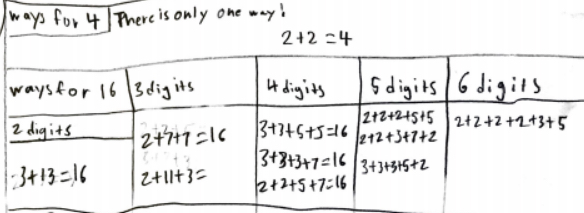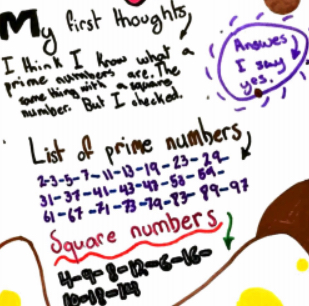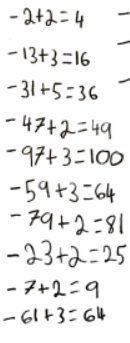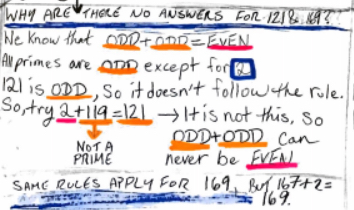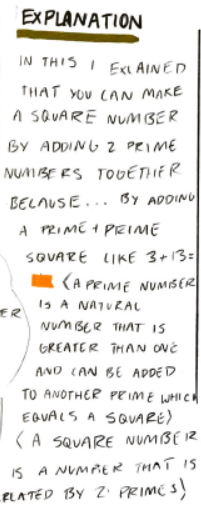Thank you for all the solutions that were submitted.